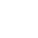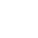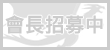LV. 17
GP 52【討論】關於在迷宮裏POWER UP所提升的攻防血

5/1/2017更新：

（*這裡攻擊力因爲武器技能的觸發變成了106）

（*這裡攻擊力因爲武器技能的觸發變成了91）

2 - 6 = +3
7 - 10 = +4
11 - 13 = +5
14 - 17 = +6
18 - 20 = +7
21 - 24  = +8
25 - 27 = +9
28 - 31 = +10
32 - 34 = +11
35 - 38 = +12
39 - 41 = +13
42 - ？？= +14
46 - ？？= +15
？？ - ？？ = +16
55 - ？？= +17
？？ - 62  = +19
63或以上 = +20

16 = +8
20 = +9
22 = +10
27 = +11
29 = +12
31 = +12
32 = +13
35 = +14
39 = +15
42 = +16
49 = +18
58 = +20
64 = +22
102 = +33
112 = +36
138 = +43
155或以上 = +44

1.攻擊力和防禦力看起來目前的增加值CAP在20，最少要63才能得到+20。
2.血量目前增加值CAP在44。我猜測大概140+左右才能得到+44。face基於日前微軟官方表示 Internet Explorer 不再支援新的網路標準，可能無法使用新的應用程式來呈現網站內容，在瀏覽器支援度及網站安全性的雙重考量下，為了讓巴友們有更好的使用體驗，巴哈姆特即將於 2019年9月2日 停止支援 Internet Explorer 瀏覽器的頁面呈現和功能。